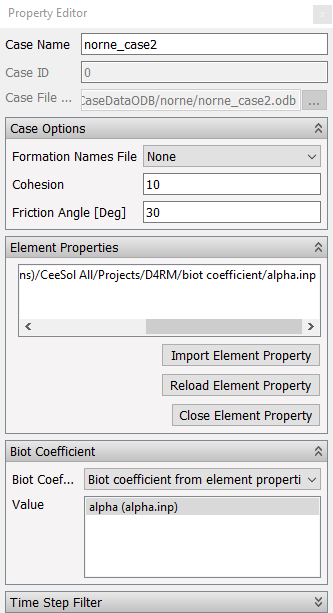# Biot Coefficient

Background

The Biot porelastic coefficient defines the compressibility of sand grains, and will influence some of the calculations in ResInsight.

## Description

The Biot coefficient can be defined in three ways:

• Default (Constant value of 1.0)
• User defined constant for all element
• One value for each element based on imported values from an element property table

These settings are controlled from the Property Editor when a Geomechanical Case is selected. To use Biot coefficients for each element, perform the following steps:

• Import values using Import Element Property
• Select Biot Coefficient from element properties
• Select the property to be used for Biot coefficient valuesElement Property Table

Derived Results